# How to Find Horizontal Asymptotes

## What is a Horizontal Asymptote

An asymptote is a line or curve that become arbitrarily close to a given curve. In another words it is a line close to a given curve, such that the distance between the curve and the line approaches zero when the curve is reaching higher/ lower values. The region of the curve that has an asymptote is asymptotic. Asymptotes are often found in rotational functions, exponential function and logarithmic functions. Asymptote parallel to the x-axis is known as a horizontal axis.

## How to Find the Horizontal Asymptote

An asymptote exists if the function of a curve is satisfying following condition. If f(x) is the curve, then a horizontal asymptote exist if ,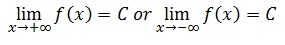Then horizontal asymptotes exist with equationy=C. If the function approaches finite value (C)at infinity, the function has an asymptote at that valueand the equation of an asymptote is y=C. A curve may intersect this line at several points, but becomes asymptotic as it approaches infinity.

To find the asymptote of a given function, find the limits at infinity.

## Finding horizontal asymptotes – Examples

• ### Exponential functions of form f(x)=ax and [a>0]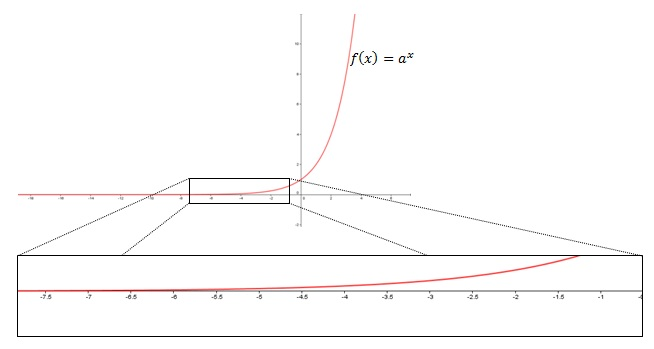Exponential functions are the simplest examples of horizontal asymptotes.

Taking the limits of the function at positive and negative infinities gives, limx→-∞ ax =+∞ and limx→-∞ ax =0. The right limit is not a finite number and tends to positive infinity, but the left limit approaches the finite values 0.

Therefore, we can say that exponential function f(x)=ax has a horizontal asymptote at 0. The equation of the asymptote line is y=0, which also is the x-axis. Since a is any positive number, we can consider this as a general result.

When a=e= 2.718281828, the function is also known as the exponential function. f(x)=ex has specific characteristics and therefore, important in mathematics.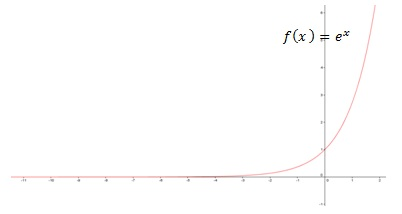• ### Rational functions

A function of the form f(x)=h(x)/g(x) where h(x),g(x) are polynomials and g(x)≠0, is known as a rational function. Rational function may have both vertical and horizontal asymptotes.

i. Consider the function f(x)=1/x

Function f(x)=1/x has both vertical and horizontal asymptotes.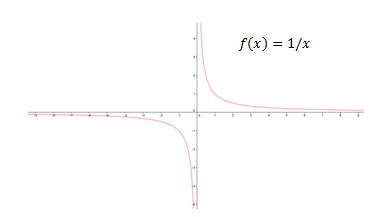To find the horizontal asymptote find the limits at infinity.
limx→=+∞ 1/x=0and limx→=-∞ 1/x =0
When x→+∞, function approaches 0 from the positive side and when x→=-∞ function approaches 0 from the negative direction.
Since function has a finite value 0 when approaching infinities, we can deduce that the asymptote is y=0.

ii. Consider the function f(x)=4x/(x2+1)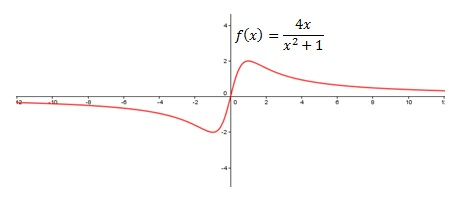Again find the limits at infinity to determine the horizontal asymptote.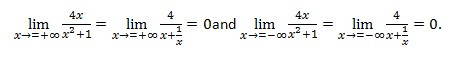Again the function has asymptote y=0, also in this case the function intersects the asymptote line at x=0

iii. Consider the function f(x)=(5x2+1)/(x2+1)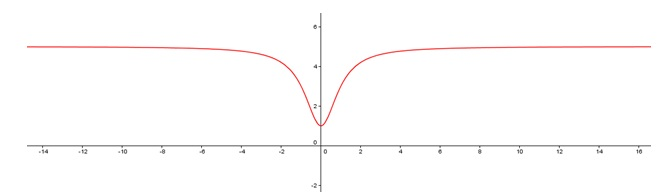Taking the limits at infinity gives,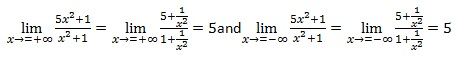Therefore, the function has finite limits at 5. So, the asymptote is y=5